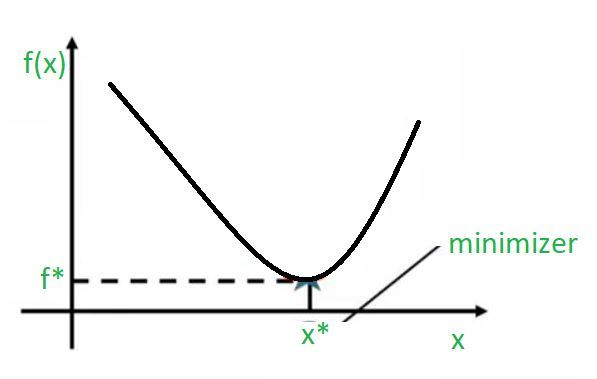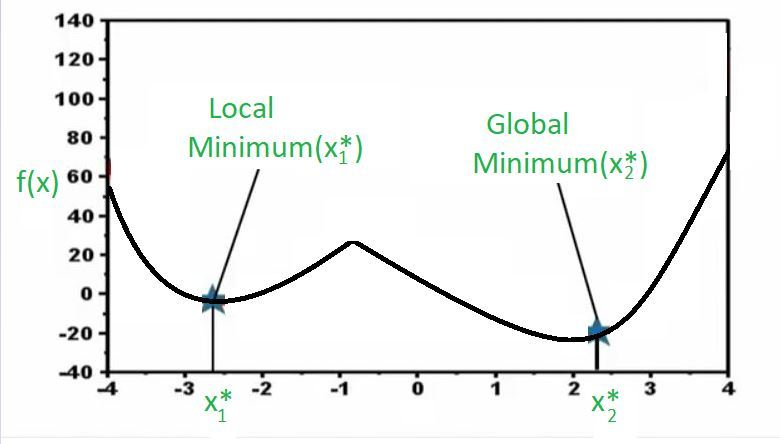Related Articles
Local and Global Optimum in Uni-variate Optimization
• Last Updated : 16 Jul, 2020

Uni-variate optimization is a simple case of a non-linear optimization problem with an unconstrained case that is there is no constraint. Uni-variate optimization may be defined as a non-linear optimization with no constraint and there is only one decision variable in this optimization that we are trying to find a value for.

min f(x) such that x ∈ R

where,
f(x) = Objective function
x = Decision variable

So, when you look at this optimization problem you typically write it in this above form where you say you are going to minimize f(x), and this function is called the objective function. And the variable that you can use to minimize this function which is called the decision variable is written below like this w.r.t x here and you also say x is continuous that is it could take any value in the real number line. And since this is a uni-variate optimization problem x is a scalar variable and not a vector variable.

Convex Function:

Whenever we talk about uni-variate optimization problems it is easy to visualize that in a 2D picture like this.So, what we have here is on the x-axis, we have different values for the decision variable x and in the y-axis, we have the function value. And when you plot this you can quite easily notice in the graph that marked the point at which this function attains its minimum value. So, the point at which this function attains minimum value can be found by dropping a perpendicular onto the x-axis. So, you can say x* is the actual value of x at which this function takes a minimum value and the value that the function takes at its minimum point can be identified by dropping this perpendicular onto the y-axis and this f* is the best value this function could possibly take. So, the functions of this type are called convex functions because there is only one minimum here. So, there is no question of multiple minima to choose from there is only one minimum here, and that is marked in the graph. So, in this case, we would say that this minimum is both a local minimum and also a global minimum. In fact, we can say it is a local minimum because in the vicinity of this point this is the best solution that you can get. And if the solution that we get in the vicinity of this point is also the best solution globally then we also call it the global minimum.

Non-convex Function:Now, take a look at the above graph. Here I have a function and again it is a univariate optimization problem. So, on the x-axis, I have different values of the decision variable and on the y-axis, we plot the function. Now, you may notice that there are two points where the function attains a minimum and you can see that when we say minimum we automatically actually only mean locally minimum because if you notice this x1* point in the graph, in the vicinity of this point, this function cannot take any better value from a minimization viewpoint. In other words, if I am at x1* and the function is taking this value, if I move to the right, the function value will increase which basically is not good for us because we are trying to find minimum value, and if I move to my left the function value will again increase which is not good because we are finding the minimum for this function. What this basically says is the following.

This says that in a local vicinity you can never find a point which is better than this. However, if you go far away then you will get to this point(x2*) here which again from a local viewpoint is the best because if we go in the right direction the function increases and if we go in the left direction also the function increases, and in this particular example it also turns out that globally this is the best solution. So, while both are local minimum in the sense that in the vicinity they are the best but this local minimum(x2*) is also global minimum because if you take the whole region you still can’t beat this solution. So, when you have a solution which is the lowest in the whole region then we call that as a global minimum. And these are types of functions that we call as non-convex functions where there are multiple local optima and the job of an optimizer is to find out the best solution from the many optimum solutions that are possible.

Why this concept is important for Data Science?

Let’s make a connection between this concept and data science. This problem of finding the global minimum has been a real issue in several data science algorithms. For example, in the 90s there was a lot of excitement and interest in neural networks and so on, and for a few years lot of research went into neural networks and in many cases, it turned out that finding the global optimum solution was very difficult and in many cases, these neural networks trained to local optima which are not good enough for the type of problems that were that being solved. So, that became a real issue with the notion of neural networks, and then in recent years this problem has been revisited and now there are much better algorithms, and much better functional forms, and much better training strategies. So that you can achieve some notion of global optimality and that is the reason why we have these algorithms make a comeback and be very useful.My Personal Notes arrow_drop_up
Recommended Articles
Page :